# Test: Mensuration, Solid Geometry Questions

## 20 Questions MCQ Test Practice Questions for GMAT | Test: Mensuration, Solid Geometry Questions

Description
Attempt Test: Mensuration, Solid Geometry Questions | 20 questions in 20 minutes | Mock test for GMAT preparation | Free important questions MCQ to study Practice Questions for GMAT for GMAT Exam | Download free PDF with solutions
QUESTION: 1

### A 5 cubic centimeter cube is painted on all its side. If it is sliced into 1 cubic centimer cubes, how many 1 cubic centimeter cubes will have exactly one of their sides painted?

Solution:

When a 5 cc cube is sliced into 1 cc cubes, we will get 5*5*5 = 125 1 cc cubes.

In each side of the larger cube, the smaller cubes on the edges will have more than one of their sides painted.
Therefore, the cubes which are not on the edge of the larger cube and that lie on the facing sides of the larger cube will have exactly one side painted.

In each face of the larger cube, there will be 5*5 = 25 cubes. Of these, there will be 16 cubes on the edge and 3*3 = 9 cubes which are not on the edge.
Therefore, there will be 9 1-cc cubes per face that will have exactly one of their sides painted.
In total, there will be 9*6 = 54 such cubes.

QUESTION: 2

### The area of a square field is 24200 sq m. How long will a lady take to cross the field diagonally at the rate of 6.6 km/hr?

Solution:

Let ‘a’ meters be the length of a side of the square field.

Therefore, its area = a2 square meters. --- (1)

We know that the length of the diagonal ‘d’ of a square whose side is ‘a’ meters = √2---(2)
From (1) and (2), we can deduce that the square of the diagonal = d2 = 2a2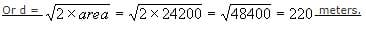The time taken to cross a length of 220 meters while traveling at 6.6 kmph is given by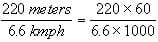(converting 1 km = 1000 meters and 1 hour = 60 minutes).

QUESTION: 3

### The circumference of the front wheel of a cart is 30 ft long and that of the back wheel is 36 ft long. What is the distance travelled by the cart, when the front wheel has done five more revolutions than the rear wheel?

Solution:

The circumference of the front wheel is 30 ft and that of the rear wheel is 36 feet.

Let the rear wheel make n revolutions. At this time, the front wheel should have made n+5 revolutions.

As both the wheels would have covered the same distance, n*36 = (n+5)*30

36n = 30n + 150

6n = 150

n = 25.

Distance covered = 25*36 = 900 ft.

QUESTION: 4

If the sides of a triangle measure 72, 75 and 21, what is the measure of its in radius?

Solution:

The sides of the triangle happen to be a Pythagorean triplet. Hence, the triangle is a right angled triangle.

For a right angled triangle,

The measure of in radius = (product of perpendicular sides) / (perimeter of triangle)

= (72 * 21) / (72 + 21 +75) = 1512 / 168 = 9

QUESTION: 5

A 5 cm cube is cut into as many 1 cm cubes as possible. What is the ratio of the surface area of the larger cube to that of the sum of the surface areas of the smaller cubes?

Solution:

The volume of the larger cube = 53 = 125 cm3.

The volume of each of the smaller cubes = 13 = 1 cm3. Therefore, one would get 125 smaller cubes.

The surface area of the larger cube = 6a2 = 6(52) = 6 * 25 = 150

The surface area of each of the smaller cubes = 6 (12) = 6.

Therefore, surface area of all of the 125, 1 cm3 cubes = 125 * 6 = 750.

Therefore, the required ratio = 150 : 750 = 1 : 5

QUESTION: 6

If each interior angle of a regular polygon is 150 degrees, then it is a/an ______________

Solution:

The sum of the exterior angle and interior angle of a regular polygon is 3600.

As the measure of each interior angle is 150, the measure of each exterior angle will be 300.

The number of sides of a regular polygon is given by the following relationship n = 360/exterior angle

As the value of each exterior angle is 300, the number of sides = 12.

QUESTION: 7

Four horses are tethered at 4 corners of a square field of side 70 metres so that they can just about reach one another. The area left ungrazed by the horses is:

Solution:

The length of the rope in which the horses tied should be equal to half of the side of the square plot so that they just cannot reach one another.

Therefore, the length of the rope is 35m (70/2).

The area covered by each horse should be equal to the area of sector with radius of 70/2 = 35m(length of the rope).

Total area covered by the four horses = 4* area of sector of radius 35 metres = Area of circle of radius 35m.

Area left ungrazed by the horses = Area of square field - Area covered by four horses.

= 702 - (22/7)*35*35 = 4900 - 3850 = 1050 sq.m.

QUESTION: 8

A square sheet of paper is converted into a cylinder by rolling it along its length. What is the ratio of the base radius to the side of the square?

Solution:

Surface area of the cylinder = Surface area of the square  2πh = a2
Here height 'h' of the cylinder = side of the square since it is rolled along its length. 2πa = a2
Base radius r = a / 2π
Ratio of base radius to side of square =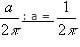QUESTION: 9

The surface area of the three coterminous faces of a cuboid are 6, 15, 10 sq.cm respectively. Find the volume of the cuboid.

Solution:

If l, b, h be the dimensions of the cuboid, then volume of the cuboid = l X b X h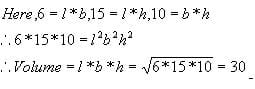QUESTION: 10

If the diagonal and the area of a rectangle are 25 m and 168 m2, what is the length of the rectangle?

Solution:

The diagonal d = 25m. and area A = 168 m2.
Let 'l' be the length and 'b' be the width of the rectangle.
Therefore, l2 + b2 = d2. and lb = A
We can therefore write (l + b)2 = d2 + 2A and (l - b)2 = d2 - 2A.
Substituting and solving we get, l + b = 31 and l - b = 17. Hence l = 24 and b = 7

QUESTION: 11

A 4 cm cube is cut into 1 cm cubes. What is the percentage increase in the surface area after cutting?

Solution:

Volume of 4 cm cube = 64 cc. When it is cut into 1 cm cube, the volume of each of the cubes = 1cc
Hence, there will be 64 such cubes. Surface area of small cubes = 6 (12) = 6 sqcm.
Therefore, the surface area of 64 such cubes = 64 * 6 = 384 sqcm.
The surface area of the large cube = 6(42) = 6*16 = 96.
% increase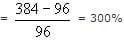QUESTION: 12

A regular hexagon is inscribed in a circle of radius r cm. What is the perimeter of the regular hexagon?

Solution:

A regular hexagon comprises 6 equilateral triangles, each of them having one of their vertices at the center of the hexagon. The sides of the equilateral triangle are equal to the radius of the smallest circle inscribing the hexagon. Hence, each of the side of the hexagon is equal to the radius of the hexagon and the perimeter of the hexagon is 6r.

QUESTION: 13

If x units are added to the length of the radius of a circle, what is the number of units by which the circumference of the circle is increased?

Solution:

Let the radius of the circle be 'r' units.
The circumference of the circle will therefore be 2πr units.
If the radius is increased by 'x' units, the new radius will be (r + x) units.

The new circumference will be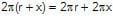Or the circumference increases by 2πx units .

QUESTION: 14

ABCD has area equal to 28. BC is parallel to AD. BA is perpendicular to AD. If BC is 6 and AD is 8, then what is CD?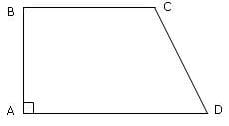Solution:

The given shape is a trapezium.
Area of a trapezium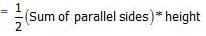28 =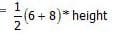Height = 4.

BA is perpendicular to BC and AD.

So, drop a line parallel to BA from C to meet AD at E.
CED is a right triangle with side CE measuring 4 and ED measuring 2 units.

Hence, CD, the measure of the hypotenuse =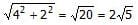QUESTION: 15

Which of the following figures has the largest area?
An equilateral triangle whose sides each have length 4
A triangle whose sides have lengths 3, 4 and 5

Solution:

Area of circle of radius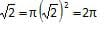Area of an equilate ral triangle whose sides each have length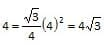Area of a triangle whose sides measure 3, 4 and 5. The given triangle is a right triangle.
Hence, its area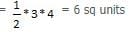We need to compare these three numbers.

Area of the circle,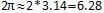6.28 sq units

Area of the equilateral triangle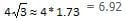Area of the right triangle = 6 sq units.

The area of the equilateral triangle is the largest amongst the 3 objects given in this question.

QUESTION: 16

The hexagon ABCDEF is regular. That means all its sides are of the same length and all its interior angles are of the same size. Each side of the hexagon is 2 m. What is the area of the rectangle BCEF?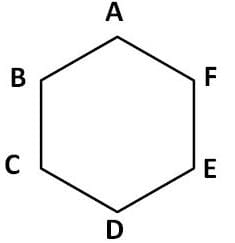Solution: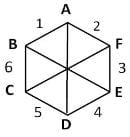A regular hexagon comprises six equilateral triangles - each of side 2 m, the measure of the side of the regular hexagon - as shown above. The 6 triangles are numbered 1 to 6 in the figure shown above.

BX is the altitude of triangle 1 and XF is the altitude of triangle 2.
Both triangle 1 and triangle 2 are equilateral triangles.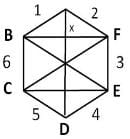Hence, BX = XF =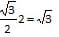Therefore, BF, the length of the rectangle = 2√3 M
Hence, the area of the rectangle BCEF = length * width =  2√3 * 2 = 4 √3 sq. m

QUESTION: 17

Ram a farmer, managed to grow shaped-watermelons inside glass cases of different shapes. The shapes he used were: a perfect cube, hemi-spherical, cuboid, cylindrical along with the normal spherical shaped watermelons. Thickness of the skin was same for all the shapes. Each of the glass cases was so designed that the total volume and the weight of the all the water-melons would be equal irrespective of the shape.
A customer wants to buy water-melon for making juice, for which the skin of the water-melon has to be peeled off, and therefore is a waste. Which shape should the customer buy?

Solution:

This is the kind of question that is either very easy or very difficult depending on whether you know the concept behind the question.

For a given surface area, the volume contained increases with increasing symmetry of the object. For instance, if we are to make water melons of different shapes of the same surface area, the volume will be maximum when it is made into a sphere.

The corollary is that for a given volume, the surface area will be minimum when the object is a sphere. So, the customer should opt for spherical shaped water melons if she has to minimize wastage.

For 2-dimensional object, for a given perimeter, the area increases with increasing symmetry.

Among different triangles of a given perimeter, an equilateral triangle has the largest area.

The area increases with increasing number of sides - i.e., for a given perimeter the area of a square will larger than that of an equilateral triangle; the area of a regular pentagon of a given perimeter will be larger than that of a square and so on.

Among different regular polygons of a given perimeter / circumference a circle has the largest area.

QUESTION: 18

The figure below has been obtained by folding a rectangle. The total area of the figure (as visible) is 144 square meters. Had the rectangle not been folded, the current overlapping part would have been a square. What would have been the total area of the original unfolded rectangle?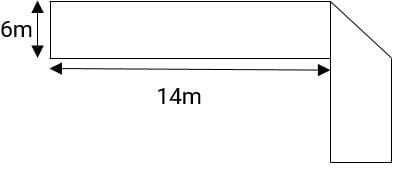Solution:

Folded part as shown in the first figure is a triangle - a right triangle.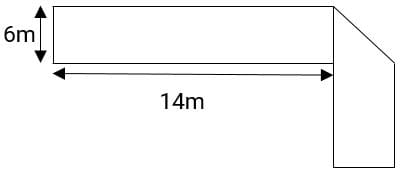The two perpendicular sides of the right triangle measure 6m each. So, the triangle is a right isosceles triangle.

When unfolded the folded area becomes a square as shown in the following figure.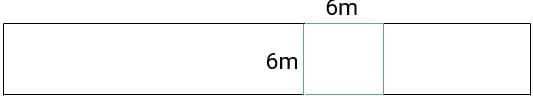The side of the square will be the width of the larger rectangle and is therefore, 6m.

Area of the square = 6 * 6 = 36 sq.m

When folded, only the area of the right triangle gets counted.
However, when unfolded the area of square gets counted.
The square comprises two congruent right triangles.

In essence, when folded only half a square is counted. When unfolded the entire square gets counted.

The area of the rectangle when unfolded = area of the rectangle when folded + area of half a square.
So area after unfolding= 144 + 18 = 162 sq.m.

QUESTION: 19

A solid metal cylinder of 10 cm height and 14 cm diameter is melted and re-cast into two cones in the proportion of 3 : 4 (volume), keeping the height 10 cm. What would be the percentage change in the flat surface area before and after?

Solution:

Change in flat surface area = 21k – 14k = 7k
% change in flat surface area  7k/14k * 100 = 50%.

QUESTION: 20

A circular road is constructed outside a square field. The perimeter of the square field is 200 ft. If the width of the road is 7√2 ft. and cost of construction is Rs. 100 per sq. ft. Find the lowest possible cost to construct 50% of the total road.

Solution:

The area of the circular road
= π ro2 - π ri2, where ro is the outer radius and ri is the inner radius.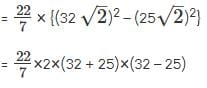25= 2508 sq. ft.

If per sq. ft. cost is Rs. 100, then cost of constructing the road = 2508 × 100 = Rs.2,50,800.

Cost of constructing 50% of the road = 50% of the total cost = 250800/2 =Rs.1,25,400Use Code STAYHOME200 and get INR 200 additional OFF Use Coupon Code